Circle it Up
Scaling it Down ... or Up
Find the X
Miscellaneous
100

What is the line that goes from the center of a circle to the outside boundary called?

100

Miss. McKinley's favorite color is ...

Blue

100

Is this picture a scale drawing? Be ready to explain your reasoning.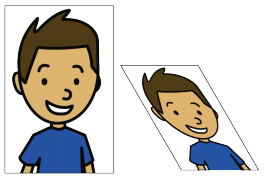No this is not a scaled drawing because the picture on the left is slanted.
100

When you have a letter in an equation what do you call it?

A variable or the unknown

100

Add the fractions 1/2 and 5/6

200

What is the outside boundary called? It means perimeter but for a circle.

The Circumference

200

Miss. McKinley's favorite food is ....

Her mom's buffalo hot wings

200

What does C.O.P stand for?

Constant of Proportionality

200

Pick one person from your team.

There is an X hidden somewhere in the room. On my mark find it!!

200

Pick one member from your team

You must balance a yard stick on your finger tips. The person with the longest time wins.

300

Find the Circumference of the circle (Round to the nearest hundredth). Use the formula

C=2pir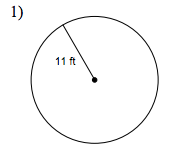C = 69.12
300

What sport does Miss. McKinley perform in and also coach?

Colorguard

300

Is this graph proportional? Be ready to explain your reasoning.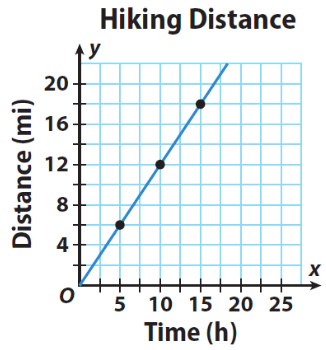Yes, because it is a straight line and it goes through the origin

300

Solve for x

3(x-10)=12

x = 14

300

Pick two players from your team.

Arrange the popsicle sticks into the correct steps to solve the equation.

400

Find the area of the circle (round to the nearest hundredth). Use the formula A=pi*r2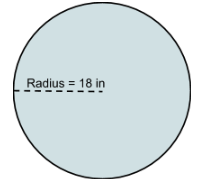A=1017.88

400

Miss. McKinley went to college at .... (spelling counts)

Quinnipiac University

400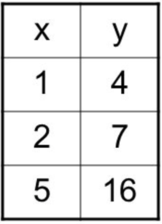No because the Constant of Proportionality or the scale factor is not the same.

400

Solve for x

x/2+4=11

x=14

400

Pick two players from your team.

You must arrange the letters to figure out the phrase.

500

Which line segment is a diameter?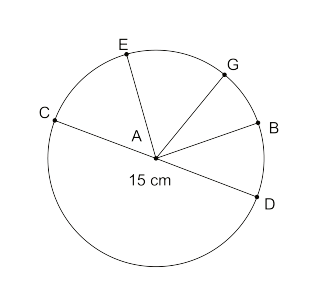Line segment CD

500

On a scale from 1-10 how much do you think Miss. McKinley is going to miss you guys?

11

500

Write an equation to represent the table below. Make sure it is in the for y = ___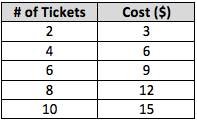The equation is ...

y=1.5x

or

y=3/2 x

500

Solve for x

-4(x+2)=19

x=6.75 or x=27/4 or x=6 3/4

500

What place value is marked off in this decimal.

235.167107

The thousandths place
Click to zoom